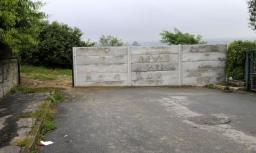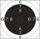# High wall

I have a wall 2m high. I need a 15 degree angle (upward) to second wall 4 meters away. How high must the second wall?

Correct result:

x =  3.07 m

#### Solution:We would be pleased if you find an error in the word problem, spelling mistakes, or inaccuracies and send it to us. Thank you!Tips to related online calculators

#### You need to know the following knowledge to solve this word math problem:

We encourage you to watch this tutorial video on this math problem:

## Next similar math problems:

• ShooterThe shooter fired to a target from distance 11 m The individual concentric circle of targets have a radius increments 1 cm (25 points) by 1 point. Shot was shifted by 8'(angle degree minutes). How many points should win his shot?
• CloudsApproximately at what height is the cloud we see under an angle of 26°10' and see the Sun at an angle of 29°15' and the shade of the cloud is 92 meters away from us?
• A boyA boy of height 1.7m is standing 30m away from flag staff on the same level ground . He observes that the angle of deviation of the top of flag staff is 30 degree. Calculate the height of flag staff.Average climb of the road is given by ratio 1:15. By what angle road average climb?
• BevelI have bevel in the ratio 1:6. What is the angle and how do I calculate it?
• RailwayRailway line had on 5.8 km segment climb 9 permille. How many meters track ascent?
• RhombusABCD is a rhombus, ABD is an equilateral triangle and AC is equal to 4. Find the area of the rhombus.
• TreeHow tall is the tree that observed in the visual angle of 52°? If I stand 5 m from the tree and eyes are two meters above the ground.
• BuildingHow high is the building that throws horizontal shadow 95.4 m long at angle 50°?
• SteepleSteeple seen from the road at an angle of 75°. When we zoom out to 25 meters, it is seen at an angle of 20°. What is high?
• ClimbRoad has climbing 1:27. How big is a angle corresponds to this climbing?
• Depth angleFrom a cliff of 150 meters high, we can see the ship at a depth angle of 9° at sea. How far is the ship from the cliff?
• ConeCalculate volume and surface area of ​​the cone with a diameter of the base d = 15 cm and side of cone with the base has angle 52°.
• Perimeter of triangleIn triangle ABC angle A is 60° angle B is 90° side size c is 15 cm. Calculate the triangle circumference.
• Isosceles triangle 8If the rate of the sides an isosceles triangle is 7:6:7, find the base angle correct to the nearest degree.
• Cube - anglesCalculate angle between the wall diagonal and cube base. Calculate the angle between the cube body diagonal and cube base.Calculate the content of a regular 15-sides polygon inscribed in a circle with radius r = 4. Express the result to two decimal places.